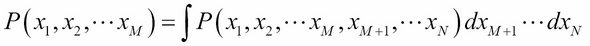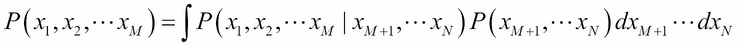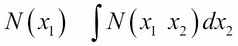•#### Learning Bayesian Models with R#### Overview of this book

Learning Bayesian Models with RCreditswww.PacktPub.comPrefaceFree Chapter
Introducing the Probability TheoryThe R EnvironmentIntroducing Bayesian InferenceMachine Learning Using Bayesian InferenceBayesian Regression ModelsBayesian Classification ModelsBayesian Models for Unsupervised LearningBayesian Neural NetworksBayesian Modeling at Big Data ScaleIndex## Marginal distribution

In many situations, we are interested only in the probability distribution of a subset of random variables. For example, in the heart disease problem mentioned in the previous section, if we want to infer the probability of people in a population having a heart disease as a function of their age only, we need to integrate out the effect of other random variables such as blood pressure and diabetes. This is called marginalization:Or:Note that marginal distribution is very different from conditional distribution. In conditional probability, we are finding the probability of a subset of random variables with values of other random variables fixed (conditioned) at a given value. In the case of marginal distribution, we are eliminating the effect of a subset of random variables by integrating them out (in the sense averaging their effect) from the joint distribution. For example, in the case of two-dimensional normal distribution, marginalization with respect to one variable will result in a one-dimensional normal distribution of the other variable, as follows:The details of this integration is given as an exercise (exercise 3 in the Exercises section of this chapter).